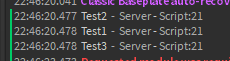# How to sort a table with other tables inside?

how would i sort a table containing other tables using table.sort?
i dont really know how to explain what i am trying to achieve so let me just give you an example:

``````local table1 = {
name="Test1",
price=50
}
local table2 = {
name="Test2",
price=5
}
local table3 = {
name="Test3",
price=500
}

local tableToSort = {table1,table2,table3}
``````

how would i use table.sort to sort these 3 tables, by the price?
desired order:
table2 (price = 5)
table1 (price = 50)
table3 (price = 500)
if anything is unclear, let me know and ill try to explain it a bit better
any help appreciated!

2 Likes
``````local table1 = {
name="Test1",
price=50
}
local table2 = {
name="Test2",
price=5
}
local table3 = {
name="Test3",
price=500
}

local tableToSort = {table1,table2,table3}

table.sort(tableToSort, function(a, b)
return a.price < b.price
end)

print(tableToSort)
``````

Not sure if this works, since I’m not good with table sorting.

10 Likes

that works, thanks for help!1 Like